# How to Multiply in Excel Using Paste Special

Watch Video – How to Multiply in Excel Using Paste Special

Excel Paste Special functionality can save a lot of time. One of its features is ‘Operations’, which allows us to quickly perform mathematical calculations (such as addition, subtraction, multiplication, and division) on a range of cells.

In this tutorial, I will show you how to multiply in Excel using paste special operators options. These come in handy when you want to avoid applying formulas or using helper columns.

Suppose you have the data set as shown below. These are sales forecast numbers, and you need to increase these forecast values by 10%.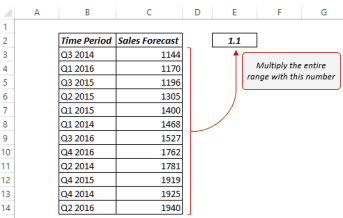One way (the long and tedious one) to do this is to use a helper column and first calculate new values by a formula, and then paste the new values in place of old values.

Another (quick and awesome) way is to use Paste Special Operation feature.

## How to Multiply in Excel Using Paste Special

Here are the steps to multiply in Excel using the Paste Special Operations:

1. Copy the value in cell E2 (1.1) [Use your favorite keyboard shortcut – Control + C].
2. Select the entire range which you need to multiply (B3:B14).
3. Go to Home –> Clipboard –> Paste –> Paste Special.
• This opens the Paste special dialogue box.
A quick way is to use the shortcut Alt + E + S.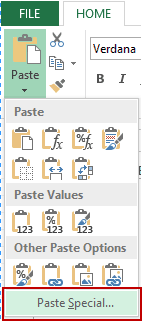4. In the Paste Special Dialogue Box, select Multiply.
Step 3 and 4 can be done by the shortcut Alt + E + S + M.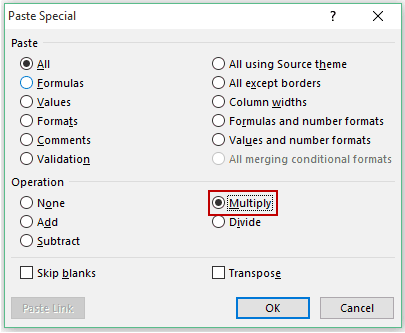5. Click OK.
6. If you are not using the value in Cell E2, you can delete it.

This will replace old numbers with new numbers.

### What If You have the Numbers as Result of a Formula

No Problem at all!! This trick works with numbers as well as formulas.

Suppose you have a formula A1+A2 in a Cell, and you want to multiply the result with a number 1.1 (which is in cell E2). Follow the same steps, as shown above, and you would get the desired result. However, note that when you use mathematical operations with a formula, it is inserted as a part of the formula (see below)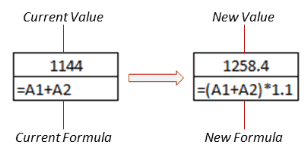### What if You want to Change every Nth Value Only?

Yes, you can!!

Suppose you want to revise the forecast numbers of Fourth Quarter Only (while the remaining numbers should be the same). This can be done using Paste Special Operation Feature with this nifty tip.

Instead of a single value, use a range of four values (as shown below):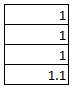Copy these four cells instead of a single cell, and follow the same steps as described above. This works by multiplying the first 3 values with 1 and the fourth with 1.1, and so on.

Cool Trick, Isn’t It!!

You May Also Like the Following Excel Tutorials: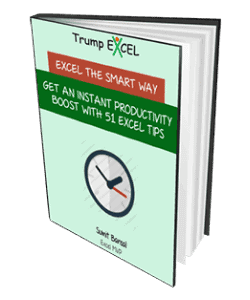FREE EXCEL BOOK

## Get 51 Excel Tips Ebook to skyrocket your productivity and get work done faster

• The Old Man, or rather Ancient One says:

I sincerely hate to spoil the party, but, by any chance, do you have the video transcribed into a text/word document? I simply do not have the time to watch a video to learn how to do something and being a long time user of Office products including Excel, there may not be much for me to learn from this and I do not mean that in any way of being mean or critisizing your work but it is just that since I have used Excel since it first came out, I may already know what is in the video and thus I do not care to watch to see if I already know it or not. Hope you understand!

• Al says:

Thank you for the Ebook!

• Me says:

Thank You!